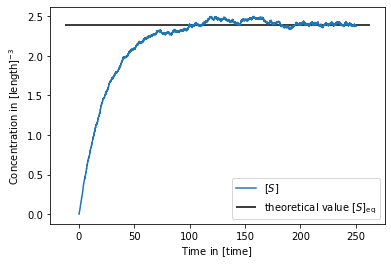# Constant concentration

This will demonstrate how to achieve a constant concentration $[S]_\mathrm{eq}$ of S particles, where the value of $[S]_\mathrm{eq}$ can be roughly adjusted under some approximations.

Define reactions of substrate with factory particles F

$$F \rightleftharpoons F + S$$

with forward propensity (intrinsic rate constant) $f_+$ and backward propensity $f_-$. The according macroscopic rates of these processes would be $k_+$ and $k_-$. In equilibrium under assumption of the law of mass action :

$$K = \frac{[F]_\mathrm{eq}[S]_\mathrm{eq}}{[F]_\mathrm{eq}} = [S]_\mathrm{eq}$$

The equilibrium constant also relates $k_+$ and $k_-$, which are functions of the propensities $f_+$ and $f_-$ respectively:

$$K = \frac{k_+(f_+)}{k_-(f_-)}$$

Since the "$+$" reaction is unimolecular we have $k_+=f_+$. The "$-$" reaction depends on the choice of reaction model. Here we choose the Doi model. Furthermore in equilibrium the effective "$-$" rate does not depend on diffusion and we can formulate it for a dilute system(well described by an isolated pair, see ) and in the absence of potentials yielding $k_-= f_- V_R$. The reaction volume is spherical $V_R=\frac{4}{3}\pi R^3$ with reaction radius $R$. Combining the equations above gives the constant concentration

$$[S]_\mathrm{eq} = \frac{f_+}{f_- V_R}$$
•  Atkins, Peter; de Paula, Julio: Atkins’ physical chemistry, Oxford University Press, 2006
•  Fröhner, Christoph; Noé, Frank: Reversible interacting-particle reaction dynamics. The Journal of Physical Chemistry B, 122.49 (2018): 11240-11250.
In :
import os
import numpy as np
import matplotlib.pyplot as plt


v2.0.2-103

In :
factory_minus_rate = 0.08
factory_plus_rate = 0.1
factory_reaction_volume = 4./3. * np.pi * factory_reaction_radius**3

concentration = factory_plus_rate / factory_minus_rate / factory_reaction_volume
print(concentration)

2.3873241463784303

In :
system = readdy.ReactionDiffusionSystem(
box_size=[10.,10.,10.],
periodic_boundary_conditions=[True, True, True],
unit_system=None
)

"factory-fusion", "F", "S", "F", factory_minus_rate,
"factory-fission", "F", "F", "S", factory_plus_rate,

In :
simulation = system.simulation("SingleCPU", reaction_handler="UncontrolledApproximation")
simulation.observe.number_of_particles(1, types=["S", "F"])
simulation.output_file = "const_concentration.h5"

if os.path.exists(simulation.output_file):
os.remove(simulation.output_file)

simulation.add_particles("F", np.random.uniform(size=(1000,3)) * 10. - 5.)

timestep = 5e-3
simulation.run(50000, timestep)

  0%|          | 9/5000 [00:00<00:58, 85.55it/s]
Configured kernel context with:
--------------------------------
- kBT = 1
- periodic b.c. = (true, true, true)
- box size = (10, 10, 10)
- particle types:
*  particle type "F" with D=1
*  particle type "S" with D=1
- unimolecular reactions:
* Fission F -> F + S with a rate of 0.1, a product distance of 0.5, and weights 0 and 1
- bimolecular reactions:
* Fusion F + S -> F with a rate of 0.08, an educt distance of 0.5, and weights 0 and 1

Configured simulation loop with:
--------------------------------
- timeStep = 0.005
- evaluateObservables = true
- progressOutputStride = 100
- context written to file = true
- Performing actions:
* Initialize neighbor list? true
* Update neighbor list? true
* Clear neighbor list? true
* Integrate diffusion? true
* Calculate forces? true
* Handle reactions? true
* Handle topology reactions? true


100%|██████████| 5000/5000 [03:09<00:00, 26.39it/s]

In :
traj = readdy.Trajectory(simulation.output_file)
plt.plot(time * timestep, counts[:,0]/volume, label=r"$[S]$")
plt.hlines(concentration, *plt.xlim(), label=r"theoretical value $[S]_\mathrm{eq}$")
plt.xlabel(r"Time in $[\mathrm{time}]$")
plt.ylabel(r"Concentration in $[\mathrm{length}]^{-3}$")